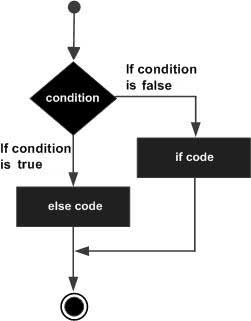# Perl UNLESS...ELSE statement

A Perl unless statement can be followed by an optional else statement, which executes when the boolean expression is true.

## Syntax

The syntax of an unless...else statement in Perl programming language is −

```unless(boolean_expression) {
# statement(s) will execute if the given condition is false
} else {
# statement(s) will execute if the given condition is true
}
```

If the boolean expression evaluates to true then the unless block of code will be executed otherwise else block of code will be executed.

The number 0, the strings '0' and "" , the empty list () , and undef are all false in a boolean context and all other values are true. Negation of a true value by ! or not returns a special false value.

## Flow Diagram## Example

```#!/usr/local/bin/perl

\$a = 100;
# check the boolean condition using unless statement
unless( \$a == 20 ) {
# if condition is false then print the following
printf "given condition is false\n";
} else {
# if condition is true then print the following
printf "given condition is true\n";
}
print "value of a is : \$a\n";

\$a = "";
# check the boolean condition using unless statement
unless( \$a ) {
# if condition is false then print the following
printf "a has a false value\n";
} else {
# if condition is true then print the following
printf "a has a true value\n";
}
print "value of a is : \$a\n";
```

When the above code is executed, it produces the following result −

```given condition is false
value of a is : 100
a has a false value
value of a is :
```
perl_conditions.htm